Mathematics
Easy

Question

# Complete the multiplication sentence.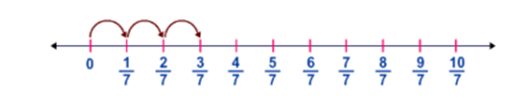3 × ? =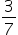.

##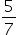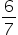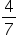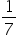Hint:

## The correct answer is:### Step by step solution:Given,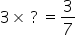Let us consider the number required to be found as 'x'.Now, the equation becomes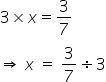Now, we all know that in fraction division, we can simply reciprocate the number to the right of the '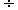' sign and replace the '' sign with 'x' sign and perform multiplication.Therefore,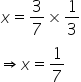Here, the 3 in the numerator of the first fraction gets reduced by the 3 in the denominator of the second fraction.Thus, we get x =which is the required answer.Hence, option (d) is the correct option.#### With Turito Foundation.#### Get an Expert Advice From Turito.